# NCERT Exemplar Notes - Class 11

## Class 11: NCERT Exemplar Notes - Class 11

The document NCERT Exemplar Notes - Class 11 is a part of Class 11 category.
All you need of Class 11 at this link: Class 11

MULTIPLE CHOICE QUESTIONS - I

Q.1. Two students performed the same experiment separately and each one of them recorded two readings of mass which are given below. Correct reading of mass is 3.0 g. On the basis of given data, mark the correct option out of the following statements.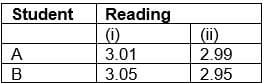(1) Results of both the students are neither accurate nor precise
(2) Results of student A are both precise and accurate
(3) Results of student B are neither precise nor accurate
(4) Results of student B are both precise and accurate
Ans.
(2)
Solution.
Average of reading of student A = 3.01 + 2.99 / 2 = 3.00
Average of readings of students B = 3.05 + 2.95 / 2 = 3.00
For both the students, average value is close to the correct value. Hence, readings of both are accurate. But readings of student A are also close to each other (differ only by 0.02) and also close to the average value, hence readings of A are also precise. But readings of B are not close to each other (differ by 0.1) and hence are not precise. Thus, results of student A are both precise and accurate.

Q.2. A measured temperature on Fahrenheit scale is 200 °F. What will this reading be on Celsius scale?
(1) 40 °C
(2) 94 °C
(3) 93.3 °C
(4) 30 °C
Ans.
(3)
Solution.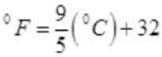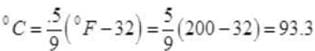Q.3. What will be the molarity of a solution, which contains 5.85 g of NaCl(s) per 500 mL?
(1) 4 mol L–1
(2) 20 mol L–1
(3) 0.2 mol L–1
(4) 2 mol L–1
Ans.
(3)
Solution.
No. of moles of NaCl = 5.85 / 58.5 = 0.1 mol/L
500 ml = 500 / 1000 = 0.5 L
Molarity (M) = No. of Mole of solute / Vol. of solution in liters
Molarity = 0.1 / 0.5 = 0.2 M

Q.4. If 500 mL of a 5M solution is diluted to 1500 mL, what will be the molarity of the solution obtained?
(1) 1.5 M
(2) 1.66 M
(3) 0.017 M
(4) 1.59 M
Ans.
(2)
Solution. M1V1 = M2V2
5 x 500 = M2 x 1500
M2 = (5 x 500) / 1500 = 1.66M

Q.5. The number of atoms present in one mole of an element is equal to Avogadro number. Which of the following element contains the greatest number of atoms?
(1) 4g He
(2) 46g Na
(3) 0.40g Ca
(4) 12g He
Ans.
(4)
Solution. 12 g He = 3 moles of He
No. of atoms of He = 3 x NA = 3 x 6.022 x 1023

Q.6. If the concentration of glucose (C6H12O6) in blood is 0.9 g L–1, what will be the molarity of glucose in blood?
(1) 5 M
(2) 50 M
(3) 0.005 M
(4) 0.5 M
Ans.
(3)
Solution.
Concentration of glucose in blood = 0.9g/L
Molar mass of glucose = 180 g mol-1
0.9g = 0.9 / 180 = 1 / 200 moles
Molarity of glucose in blood = 0.005 M

Q.7. What will be the molality of the solution containing 18.25 g of HCl gas in 500 g of water?
(1) 0.1 m
(2) 1 M
(3) 0.5 m
(4) 1 m
Ans.
(d)
Solution.
No. of moles of HCl = 18.25 / 36.5 = 0.5
Molality (m) = No. of moles in solute / Mass of solvent in Kg
Molaity = (0.5 x 1000) / 500 = 1 m

Q.8. One mole of any substance contains 6.022 × 1023 atoms/molecules.
Number of molecules of H2SO4 present in 100 mL of 0.02M H2SO4 solution is ______.
(1) 12.044 × 1020 molecules
(2) 6.022 × 1023 molecules
(3) 1 × 1023 molecules
(4) 12.044 × 1023 molecules
Ans.
(1)
Solution.

Molarity = 0.02 M, Volume of sol.= 100 mL= 0.1 L
No. of moles of H2SO4 = Molarity ∗ volume in liters
= 0.02 x 0.1 = 2 x 10-3
No. of molecules of H2SO4 = 2 x 10-3 x 6.022 x 1023
= 12.044 x 1020 molecules

Q.9. What is the mass percent of carbon in carbon dioxide?
(1) 0.034%
(2) 27.27%
(3) 3.4%
(4) 28.7%
Ans.
(2)
Solution.
Molecular mass of CO2 =1 × 12 + 2 × 16 = 44 g
1 g molecule of CO2 contains 1 g atom of carbon
44 g of CO2 contains C = 12 g of carbon
% of C in CO2 = 12 / 44 x 100 = 27.27%
Hence, the mass per cent of carbon in C02 is 27.27%

Q.10. The empirical formula and molecular mass of a compound are CH2O and 180 g respectively. What will be the molecular formula of the compound?
(1) C9H18O9
(2) CH2O
(3) C6H12O6
(4) C2H4O2
Ans.
(3)
Solution.
Empirical formula = CH2O
Empirical formula mass= 12 + 1 + 1 + 16 = 30g
Molecular mass=180 g
n = (Molar mass / Empirical formula mass) = 180 / 30 = 6
∴ n = 6
So, molecular formula = n x CH2O = 6 x CH2O = C6H12O6

Q.11. If the density of a solution is 3.12 g mL–1, the mass of 1.5 mL solution in significant figures is _______.
(1) 4.7g
(2) 4680 × 10–3g
(3) 4.680g
(4) 46.80g
Ans.
(1)
Solution.
Density of solution = 3.12 g mL-1
Volume of solution =1.5 mL
Mass of solution = Volume x Density
= 1.5 mL x 3.12 g mL-1
= 4.68 g = 4.7 g (up to 2 significant figures)

Q.12. Which of the following statements about a compound is incorrect?
(1) A molecule of a compound has atoms of different elements
(2) A compound cannot be separated into its constituent elements by physical methods of separation
(3) A compound retains the physical properties of its constituent elements
(4) The ratio of atoms of different elements in a compound is fixed
Ans.
(3)
Solution. A compound does not retain the physical or chemical properties of its constituent elements.

Q.13. Which of the following statements is correct about the reaction given below: 4Fe(s) + 3O2(g) → 2Fe2O3(g)
(1) Total mass of iron and oxygen in reactants = total mass of iron and oxygen in product therefore it follows law of conservation of mass.
(2) Total mass of reactants = total mass of product; therefore, law of multiple proportions is followed.
(3) Amount of Fe2O3 can be increased by taking any one of the reactants (iron or oxygen) in excess.
(4) Amount of Fe2O3 produced will decrease if the amount of any one of the reactants (iron or oxygen) is taken in excess
Ans.
(1)
Solution. 4Fe + 302 → 2Fe203 follows law of conservation of mass since mass of reactants is equal to mass of products.

Q.14. Which of the following reactions is not correct according to the law of conservation of mass.
(1) 2Mg(s) + O2(g) → 2MgO(s)
(2) C3H8(g) + O2(g) → CO2(g) + H2O(g)
(3) P4(s) + 5O2(g) → P4O10(s)
(4) CH4(g) + 2O2(g) → CO2(g) + 2H2O (g)
Ans.
(2)
Solution. No. of atoms in the reactant side is not equal to the no. of atoms in the product side.

Q.15. Which of the following statements indicates that law of multiple proportion is being followed.
(1) Sample of carbon dioxide taken from any source will always have carbon and oxygen in the ratio 1 : 2.
(2) Carbon forms two oxides namely CO2 and CO, where masses of oxygen which combine with fixed mass of carbon are in the simple ratio 2 : 1.
(3) When magnesium burns in oxygen, the amount of magnesium taken for the reaction is equal to the amount of magnesium in magnesium oxide formed.
(4) At constant temperature and pressure 200 mL of hydrogen will combine with 100 mL oxygen to produce 200 mL of water vapour.

Ans. (2)
Solution.
The element, carbon, combines with oxygen to form two compounds, namely, carbon dioxide and carbon monoxide. In C02, 12 parts by mass of carbon combine with 32 parts by mass of oxygen while in CO, 12 parts by mass of carbon combine with 16 parts by mass of oxygen.
Therefore, the masses of oxygen combine with a fixed mass of carbon (12 parts) in C02 and CO are 32 and 16 respectively. These masses of oxygen bear a simple ratio of 32 : 16 or 2 : 1 to each other.
This is an example of law of multiple proportion.

MULTIPLE CHOICE QUESTIONS - II

In the following questions two or more options may be correct.
Q.16. One mole of oxygen gas at STP is equal to _______.
(1) 6.022 × 1023 molecules of oxygen
(2) 6.022 × 1023 atoms of oxygen
(3) 16 g of oxygen
(4) 32 g of oxygen
Ans.
(1, 4)
Solution. 1 mole of 02 gas at STP = 6.022 x 1023 molecules of 02 (Avogadro number) = 32 g of 02. Hence, 1 mole of oxygen gas is equal to molecular weight of oxygen as well as Avogadro number.

Q.17. Sulphuric acid reacts with sodium hydroxide as follows :
H2SO4 + 2NaOH → Na2SO4 + 2H2O
When 1 L of 0.1M sulphuric acid solution is allowed to react with 1 L of 0.1 M sodium hydroxide solution, the amount of sodium sulphate formed and its molarity in the solution obtained is
(1) 0.1 mol L–1
(2) 7.10 g
(3) 0.025 mol L–1
(4) 3.55 g
Ans.
(2, 3)
Solution.
Moles of H2SO4 taken = 0.1 moles
Moles of NaOH taken = 0.1 moles
As H2SO4 and NaOH react in ratio 1 : 2, so 0.1 moles of H2SO4 reacts with 0.2 mole of NaOH which we don’t have.
0.1 mole of NaOH reacts with 0.05 mole of H2SO4, so NaOH is Limiting reactant.
Product is calculated w.r.t limiting reactant so Number of moles of Na2SO4 formed will also be equal to 0.05.
Mass of Na2S04 = 0.05 x 142 = 7.1 g
Molarity of Na2So4 = Number of moles/ volume in Litre
= 0.05 / 2 = 0.025 mol L-1

Q.18. Which of the following pairs have the same number of atoms?
(1) 16 g of O2(g) and 4 g of H2(g)
(2) 16 g of O2 and 44 g of CO2
(3) 28 g of N2 and 32 g of O2
(4) 12 g of C(s) and 23 g of Na(s)
Ans.
(3, 4)
Solution.
One mole is the amount of a substance that contains as many particles or entities as there are atoms in exactly 12 g (or 0.012 kg) of the 12 C isotope.
12g C = 1 mole of C and, and 23g Na = 1 mole of Na
28g N2 = 1 mole of nitrogen= 2 x 6.022 x 1023 atoms of nitrogen
32g O2 =1 mole of oxygen = 2 x 6.022 x 1023 atoms of oxygen

Q.19. Which of the following solutions have the same concentration?
(1) 20 g of NaOH in 200 mL of solution
(2) 0.5 mol of KCl in 200 mL of solution
(3) 40 g of NaOH in 100 mL of solution
(4) 20 g of KOH in 200 mL of solution
Ans.
(1, 2)
Solution. 20 g NaOH = 20 / 40 = 0.5 mol NaOH in 200 ml solution.
(2) Molar concentration of NaOH = 20 / 40 = 0.500 mol / 0.200L = 2.5 M
Molar concentration of KCl = 0.5 mol / 0.200L = 2.5 M

Q. 20. 16 g of oxygen has same number of molecules as in
(1) 16 g of CO
(2) 28 g of N2
(3) 14 g of N2
(4) 1.0 g of H2
Ans.
(3, 4)
Solution.
(1) Number of molecules of oxygen in 16g of oxygen = 16 / 32 x 6.023 x 1023 = 0.5 x 6.023 x 1023
(2) 0.5 moles is present in 14g of nitrogen and in 1.0 g H2. Hence they will also have 0.5 x 6.023 x 1023
(3) Number of molecules of N2 = 14 / 28 x 6.023 x 1023 = 0.5 x 6.023 x 1023
(4) Number of molecules of H2 = 1 / 2 x 6.023 x 1023 = 0.5 x 6.023 x 1023

Q.21. Which of the following terms are unitless?
(1) Molality
(2) Molarity
(3) Mole fraction
(4) Mass percent
Ans.
(3, 4)
Solution.
Mass percent = (Mass of solute / Mass of solution) x 100
Mole Fraction : It is the ratio of number of moles of a particular component to the total number of moles of the solution.

Q.22. One of the statements of Dalton’s atomic theory is given below: “Compounds are formed when atoms of different elements combine in a fixed ratio” Which of the following laws is not related to this statement?
(1) Law of conservation of mass
(2) Law of definite proportions
(3) Law of multiple proportions
Ans.
(1, 4)
Solution.
Law of conservation of mass states that matter can neither be created nor destroyed. Avogadro proposed that equal volumes of gases at the same temperature and pressure should contain equal number of molecules.

Q.23. What will be the mass of one atom of C-12 in grams?
Ans. Mass of 6.022 × 1023 atoms of C in gm = 12g
Mass of one atom of C = 12 / 6.022 x 1023 = 1.992648 x 10-23g

Q. 24. How many significant figures should be present in the answer of the following calculations?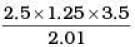Ans.= 5.4415422885
Significant figures = 2

Q.25. What is the symbol for SI unit of mole? How is the mole defined?
Ans. Symbol for SI Unit of mole is mol. One mole is defined as the amount of a substance that contains as many particles or entities as there are atoms in exactly 12 g (0.012 kg) of the 12 C isotope.

Q.26. What is the difference between molality and molarity?
Ans. Molarity : It is the most widely used unit and is denoted by M. It is defined as the number of moles of solute present in 1 litre of the solution.
Thus, Molarity (M) = No. of moles of solute / Volume of solution in litres
Molality : It is defined as the number of moles of solute present in 1 kg of solvent. It is denoted by m.
Thus, Molality (m) = No. of moles of solute / Mass of solvent in Kg
Molality is independent of temperature whereas molarity depends on temperature.

Q.27. Calculate the mass percent of calcium, phosphorus and oxygen in calcium phosphate Ca3(PO4)2.
Ans.
Molecular mass of Ca3(PO4) = 3 x 40 + 2 x 31 + 8 x 16 = 310
Mass per cent of Ca = ((3 x 40) / 310) x 100 = 38.71%
Mass per cent of P = (( 2 x 31) / 310) x 100 = 20%
Mass per cent of O = ((8 x 16) / 310) x 100 = 41.29 %

Q.28. 45.4 L of dinitrogen reacted with 22.7 L of dioxygen and 45.4 L of nitrous oxide was formed. The reaction is given below:
2N2(g) + O2(g) → 2N2O(g)
Which law is being obeyed in this experiment? Write the statement of the law
Ans.
Gay Lussac’s Law of Gaseous Volumes (Given by Gay Lussac in 1808) is being obeyed in this experiment. According to this law, when gases combine or are produced in a chemical reaction they do so in a simple ratio by volume, provided all the gases are at same temperature and pressure.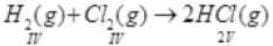(All reactants and products have simple ratio 1 : 1 : 2)

Q.29. If two elements can combine to form more than one compound, the masses of one element that combine with a fixed mass of the other element, are in whole number ratio.
(a) Is this statement true?
(b) If yes, according to which law?
(c) Give one example related to this law
Ans.
(a) Yes, the given statement is true.
(b) According to the Law of multiple proportions
(c) For example; carbon combines with oxygen to form two compounds CO and
CO2.
Masses of oxygen, which combine with a fixed mass of carbon (12 g) bear a simple ratio i.e., 16 : 32 or 1 : 2

Q.30. Calculate the average atomic mass of hydrogen using the following data: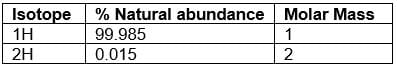Ans.
Average Atomic mass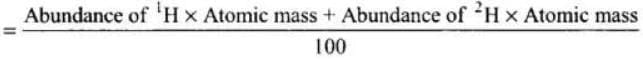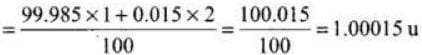Q.31. Hydrogen gas is prepared in the laboratory by reacting dilute HCl with granulated zinc. Following reaction takes place.
Zn + 2HCl → ZnCl2 + H2
Calculate the volume of hydrogen gas liberated at STP when 32.65 g of zinc reacts with HCl. 1 mol of a gas occupies 22.7 L volume at STP; atomic mass of Zn = 65.3 u.
Ans.
65.3 g of Zn reacts with HCl to form 22.7 of H2(g)
∴ 32.65 g of Zn at STP reacts with HCl to form = (22.7 x 32.65) / 65.3 = 11.35L

Q.32. The density of 3 molal solution of NaOH is 1.110 g mL–1. Calculate the molarity of the solution.
Ans.
3 molal solution of NaOH means that 3 mols of NaOH are dissolved in 1000 g of solvent.
∴ Mass of Solution = Mass of Solvent + Mass of Solute
= 1000 g + (3 × 40 g) = 1120 g
Volume of Solution = Mass / Density = 1120 / 1.110 mL = 1009.00 mL
(Since density of solution = 1.110 g mL–1)
Since 1009 mL solution contains 3 mols of NaOH
∴ Molarity (M) = No. of moles of solute / Volume of solution in litres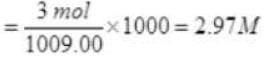Q.33. Volume of a solution changes with change in temperature, then, will the molality of the solution be affected by temperature? Give reason for your answer.
Ans. No, molality of a solution does not change with temperature since mass remains unaffected by temperature.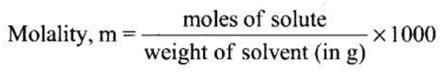Q.34. If 4 g of NaOH dissolves in 36 g of H2O, calculate the mole fraction of each component in the solution. Also, determine the molarity of solution (specific gravity of solution is 1g mL–1).
Ans.
Mole fraction of H2O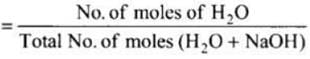No. of moles of H2O = 36 / 18 = 2 moles
No. of moles of NaOH = 4 / 40 = 0.1 mol
Total number of moles = 2 + 0.1 = 2.1
Mole fraction of H2O = 2 / 2.1 = 0.952
Mole of NaOH = 0.1 / 2.1 = 0.048
Mass of solution = Mass of H2O + Mass of NaOH
= 36 + 4 = 40 g
Volume of solution = 40 / 1 = 40 mL

Q.35. The reactant which is entirely consumed in reaction is known as limiting reagent.
In the reaction 2A + 4B → 3C + 4D, when 5 moles of A react with 6 moles of B, then
(i) which is the limiting reagent?
(ii) calculate the amount of C formed?
Ans.
2A + 4B → 3C + 4D
According to the above equation, 2 mols of ‘A’ require 4 mols of ‘B’ for the reaction.
(i) Hence, for 5 mols of ‘A’, the moles of ‘B’ required =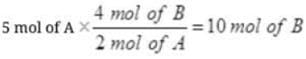But we have only 6 mols of ‘B’, hence, ‘B’ is the limiting reagent
(ii) Amount of ‘C’ formed is determined by amount of ‘B’.
Since 4 mols of ‘B’ give 3 mols of ‘C’. Hence 6 mols of ‘B’ will give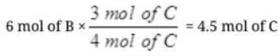MATCHING TYPE

Q.36. Match the following: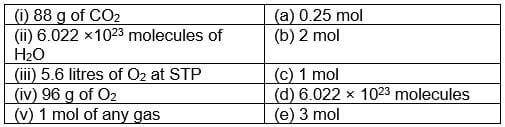Ans.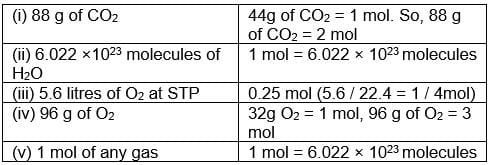Q.37. Match the following physical quantities with units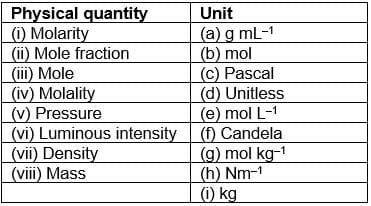Ans.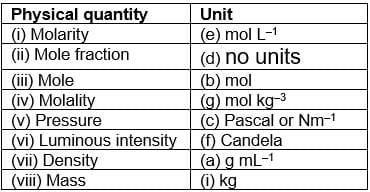ASSERTION AND REASON TYPE

In the following questions a statement of Assertion (A) followed by a statement of Reason (R) is given. Choose the correct option out of the choices given below each question.
Q.38. Assertion (A) : The empirical mass of ethene is half of its molecular mass.
Reason (R) : The empirical formula represents the simplest whole number ratio of various atoms present in a compound.
(1) Both A and R are true and R is the correct explanation of A
(2) A is true but R is false
(3) A is false but R is true
(4) Both A and R are false
Ans. (1)
Solution. Molecular formula = n x (empirical formula)
n = Molecular Mass/Empirical formula mass
Empirical formula of ethene = CH2
Empirical formula mass of ethene = 14 amu
= 1 / 2 x molecular mass of ethene

Empirical formula shows that ethene has (C : H) 1 : 2

Q 39. Assertion (A) : One atomic mass unit is defined as one twelfth of the mass of one carbon-12 atom.
Reason (R) : Carbon-12 isotope is the most abundant isotope of carbon and has been chosen as standard.
(1) Both A and R are true and R is the correct explanation of A
(2) Both A and R are true but R is not the correct explanation of A
(3) A is true but R is false
(4) Both A and R are false
Ans.
(2)
Solution. Carbon-12 is one of the isotopes of carbon and can be represented as 12C. In this system, 12C is assigned a mass of exactly 12 atomic mass unit (amu) and mass of all other atoms are given relative to this standard. One atomic mass unit is defined as a mass exactly equal to one twelfth the mass of one carbon-12 atom.

Q 40. Assertion (A) : Significant figures for 0.200 is 3 where as for 200 it is 1 Reason (R) : Zero at the end or right of a number are significant provided they are not on the right side of the decimal point
(1) Both A and R are true and R is correct explanation of A
(2) Both A and R are true but R is not a correct explanation of A
(3) A is true but R is false
(4) Both A and R are false
Ans.
(3)
Solution. Significant figures for 0.200 = 3
Significant figure for 200 = 1
Zeros at the end of a number without decimal - point, may or may not be significant depending on the accuracy of measurement.

Q.41. Assertion (A) : Combustion of 16 g of methane gives 18 g of water.
Reason (R) : In the combustion of methane, water is one of the products.
(1) Both A and R are true but R is not the correct explanation of A
(2) A is true but R is false
(3) A is false but R is true
(4) Both A and R are false

Ans. (3)
Solution. CH4(g) + 2O2(g) → CO2(g) + 2H2O(g) 16 g of CH4 on complete combustion will give 36 g of water

Q.42. A vessel contains 1.6 g of dioxygen at STP (273.15K, 1 atm pressure). The gas is now transferred to another vessel at constant temperature, where pressure becomes half of the original pressure. Calculate
(i) volume of the new vessel
(ii) number of molecules of dioxygen
Ans.
p1 = 1 atm, T1 = 273 K, V1 = ?
32 g of oxygen occupies 22.4 L of volume at STP*
Hence, 1.6 g of O2 will occupy,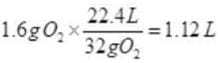V1 = 1.12 L
P2 = P1 / 2 = 1 / 2 = 0.5atm
V2 = ?
P1V1 = P2V2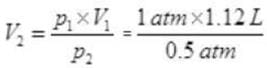= 2.24 L
(ii) Number of molecules of O2 in the vessel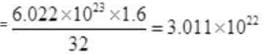Q.43. Calcium carbonate reacts with aqueous HCl to give CaCl2 and CO2 according to the reaction given below:
CaCO3 (s) + 2HCl (aq) → CaCl2(aq) + CO2(g) + H2O(l)
What mass of CaCl2 will be formed when 250 mL of 0.76 M HCl reacts with 1000 g of CaCO3? Name the limiting reagent. Calculate the number of moles of CaCl2 formed in the reaction.
Ans.
Number of moles of HCl =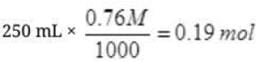Mass of CaCO3 = 1000 g
Number of moles of CaCO3 = 1000g / 100g = 10mol
According to given equation 1 mol of CaCO3(s) requires 2 mol of HCl (aq).

Hence, for the reaction of 10 mol of CaCO3(s) number of moles of HCl required would be :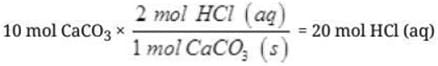But we have only 0.19 mol HCl (aq), hence, HCl (aq) is limiting reagent. So, amount of
CaCl2 formed will depend on the amount of HCl available. Since, 2 mol HCl (aq) forms 1 mol of CaCl2, therefore, 0.19 mol of HCl (aq)would give :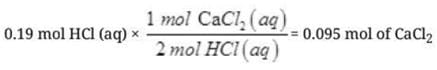Or 0.095 × molar mass of CaCl2 = 0.095 × 111 = 10.54 g

Q.44. Define the law of multiple proportions. Explain it with two examples.
How does this law point to the existence of atoms?
Ans.
The law of multiple proportions states that, “If two elements can combine to form more than one compound, the mass of one element that combine with a fixed mass of the other element, are in the ratio of small whole numbers.” For example, hydrogen combines with oxygen to form two compounds, namely, water and hydrogen peroxide.
Hydrogen + Oxygen → Water
2g             16g           18g
Hydrogen + Oxygen → Hydrogen Peroxide
2g              32g            34g
Here, the masses of oxygen (i.e. 16 g and 32 g) which combine with a fixed mass of hydrogen (2g) bear a simple ratio, i.e., 16 : 32 or 1 : 2.
This law shows that there are constituents which combine in a definate proportion. These constituents are atoms.

Q.45. A box contains some identical red coloured balls, labelled as A, each weighing 2 grams. Another box contains identical blue coloured balls, labelled as B, each weighing 5 grams. Consider the combinations AB, AB2, A2B and A2B3 and show that law of multiple proportions is applicable.
Ans. According to the law of multiple proportions, when two elements combine to form two or more compounds, then the different masses of one element, which combine with a fixed mass of the other, bear a simple ratio to one another.
For the combination, AB
1g of A combines with 5 / 2 g of B = 2.5 g B
For AB2
1g of A combines with 10 / 2 g of B = 5 g of B
For A2B
1g of A combines with 5 / 4 g of B = 1.25 g of B
For A2B2
1g of A combines with 15 / 4 g of B = 3.75 g of B
Thus, it is proved that law of multiple proportions is applicable.

The document NCERT Exemplar Notes - Class 11 is a part of Class 11 category.
All you need of Class 11 at this link: Class 11Use Code STAYHOME200 and get INR 200 additional OFF

Track your progress, build streaks, highlight & save important lessons and more!

,

,

,

,

,

,

,

,

,

,

,

,

,

,

,

,

,

,

,

,

,

;